Next: References Up: Computer Vision IT412 Previous: Fourier transform theory

# The discrete Fourier transform

So far, we have been considering functions defined on the continuous line. In digital images we can only process a function defined on a discrete set of points. This leads us to the discrete Fourier transform(DFT), whose equations are very similar to those for the continuous Fourier transform.

The DFT provides information over a discrete number of frequencies, so we need to determine precisely which frequencies these are. To do this, we sample a continuous function f at intervals of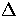, generating a sequence of sampled values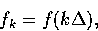where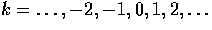The reciprocal ofis the sampling rate, or frequency. For any sampling interval, there is a special frequency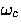called the Nyquist critical frequency: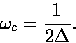The Nyquist frequency represents the highest frequency that can be represented by something sampled at intervals of, that is, a frequency having wavelength of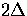. Alternatively, if one is seeking to describe a function by a set of discrete values, we must sample the function at 2 times the highest frequency in the function.

For example, critical sampling of a sine wave is at 2 sample points per cycle; sampling at any slower rate will miss'' the sine wave.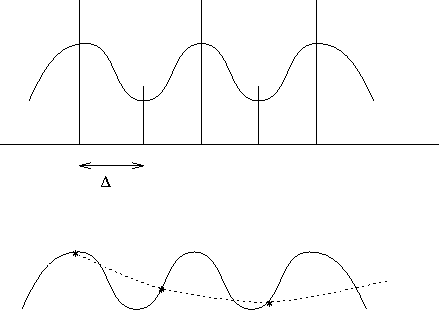If we wish to take the Fourier transform of N sampled values of a function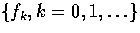with N items of input, then we will not be able to produce more than N independent items of output.

We generate the Fourier transform at discrete frequencies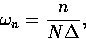where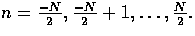These limits correspond to the upper and lower Nyquist frequencies.

We now approximate the integral equation for the Fourier transform by a discrete summation: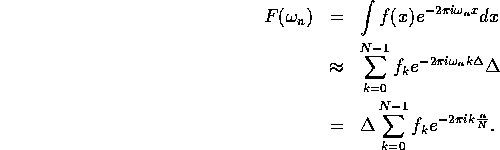Typically, the sampling distanceis set to 1, so the discrete Fourier transform is given by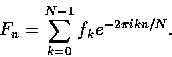The inverse discrete Fourier transform is given by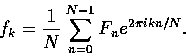Note that the only differences between the forward and inverse transforms are (i) changing the sign in the exponential, and (ii) dividing the answer by N.

So far, we are considering n to vary from -N/2 to N/2 as k varies from 0 to N-1. However, our equation for the DFT is periodic in n with period N, that is,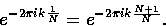Thus, if we let n vary from 0 to N-1 (which is a complete period) we will cover all the frequencies and n will vary in exact correspondence to k. Thus
• n = 0 corresponds to the zero frequency component,
•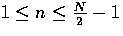corresponds to the frequencies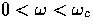,
•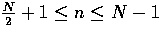corresponds to the frequencies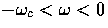, and
• n = N/2 corresponds to both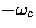and.
All standard implementations of the FFT produce data in this form.

The DFT needs N complex multiplications of fk by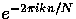and N-1 additions of the resulting values to transform N values; its complexity is thus proportional to N2, thus justifying its nickname of the Slow Fourier Transform.

The Fast Fourier Transform (FFT) is an ingenious algorithm which exploits various properties of the Fourier transform to enable the transformation to be done in O(N log2 N) operations. However, the FFT requires the size of the input data to be a power of 2; if this is not the case, the data are either truncated or padded out with zeros.Next: References Up: Computer Vision IT412 Previous: Fourier transform theory
Robyn Owens
10/29/1997